The evaluate action is very helpful when you are computing an integral which is composed of multiple integrals or when integrals are mixed with other operations such as when you need to take the limit to compute an improper integral.

• In the examples below we need to compute multiple integrals, which we can possibly type what each of the integral equals to in the next cell or use the "Evaluate" action to compute them one at a time as a subproblem. After selecting one of the integrals, select "Evaluate" action to open a sub-window to compute the selected integral.  Note that "Evaluate" action for integrals works very similar to "Compute" action for derivatives.

Example of an indefinite integral: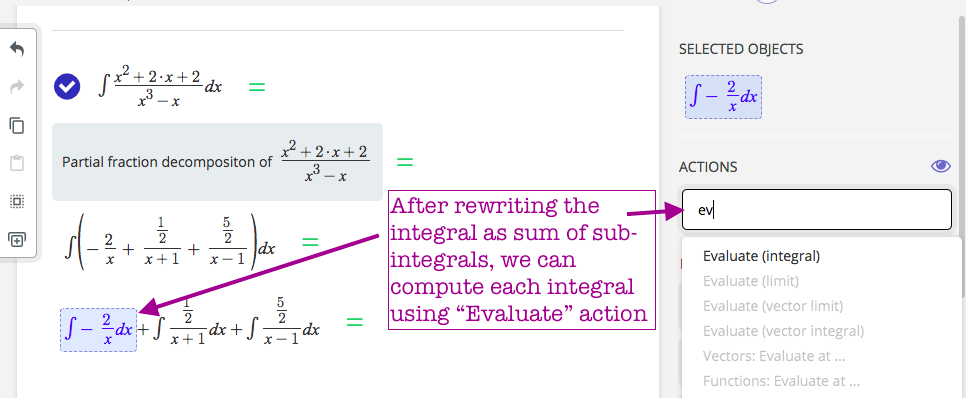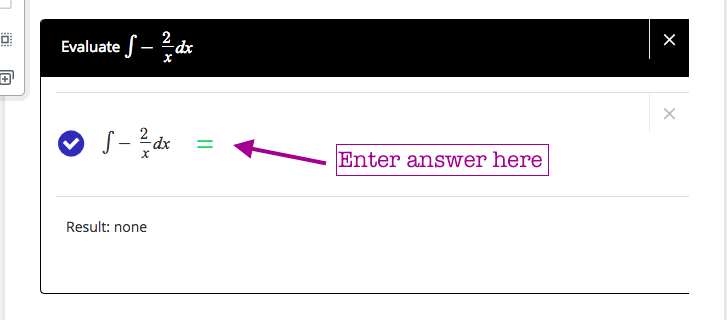REMARK: Note that you can use any integration methods you need to compute this integral: Just search for "integral" in the search box to find out all available actions to choose from.• Your result will place the sub-integral you have computed in the original integral. Now repeat the same process for all other sub-integrals to obtain the final answer for the integral we started with.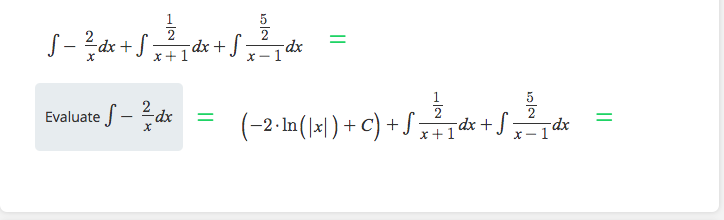Example of a definite integral:

Similarly we will use "Evaluate (integrals)" action to compute an integral as a sub problem: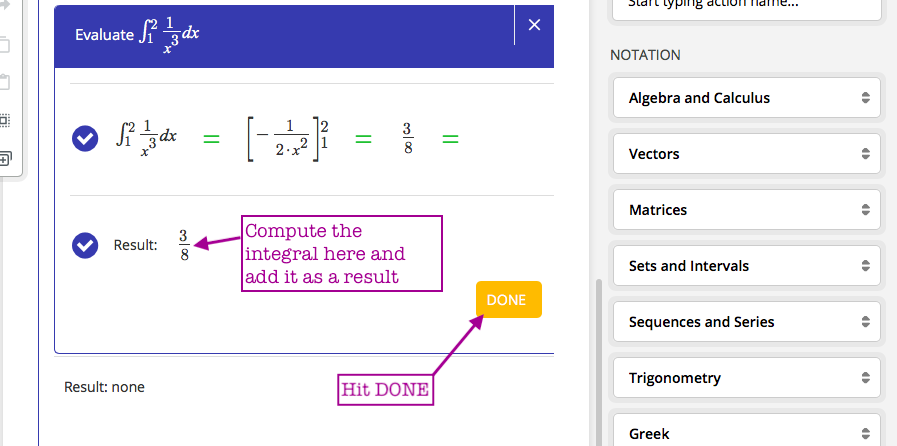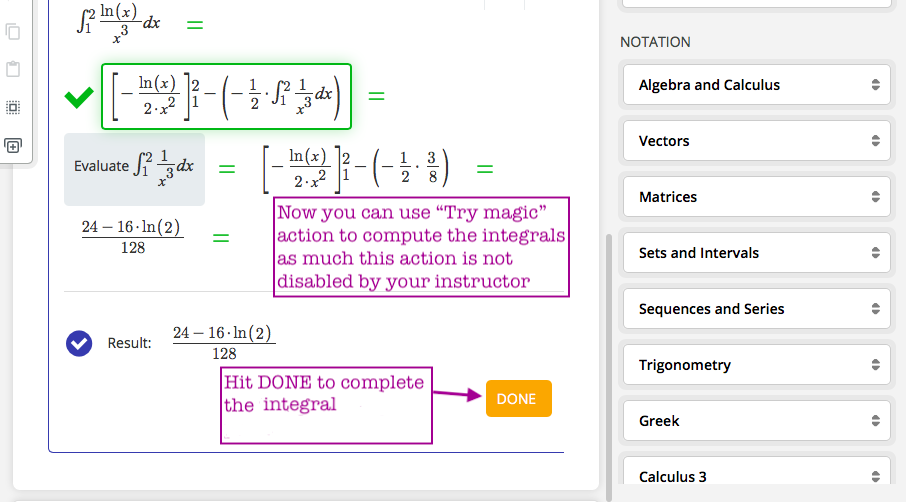Use this action whenever you need to compute integrals as sub-problems.

Hope you have found this helpful. In case you have questions or concerns on using "Integrals: Evaluate" action do not hesitate to contact us here.